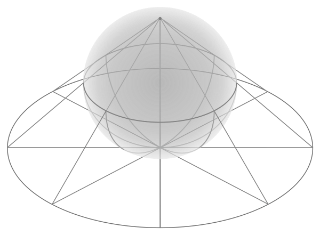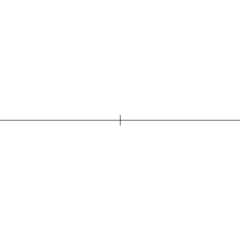# Euclidean geometry

Topic | v1 | created by janarez |Description

Euclidean geometry is a mathematical system attributed to Alexandrian Greek mathematician Euclid, which he described in his textbook on geometry: the Elements. Euclid's method consists in assuming a small set of intuitively appealing axioms, and deducing many other propositions (theorems) from these. Although many of Euclid's results had been stated by earlier mathematicians, Euclid was the first to show how these propositions could fit into a comprehensive deductive and logical system. The Elements begins with plane geometry, still taught in secondary school (high school) as the first axiomatic system and the first examples of mathematical proofs. It goes on to the solid geometry of three dimensions. Much of the Elements states results of what are now called algebra and number theory, explained in geometrical language.

##### Relations

In mathematics, a conic section (or simply conic) is a curve obtained as the intersection of the surf...Geometry (from the Ancient Greek: γεωμετρία; geo- "earth", -metron "measurement") is, with arithmetic...Pts is lightweight and modular modern javascript library. Written in typescript, it comes with many u...Straightedge and compass construction, also known as ruler-and-compass construction or classical cons...